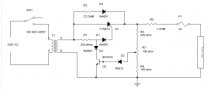# Automatic car battery charger circuit diagramThe battery is charged with small amount of AC voltage or DC voltage. So if you want to charge your battery with AC source then should follow these steps, we need first limit the large AC voltage, need to filter the AC voltage to remove the noise, regulate and get the constant voltage and then give the resulting voltage to the battery for charging. Once charging is completed the circuit should automatically turned off.

The AC source is given to the step down transformer which converts the large AC source into limited AC source, filter the AC voltage and remove the noise and then give that voltage to the SCR where it will rectify the AC and give the resulting voltage to the battery for charging.

#### Circuit Diagram of Battery Charger Using SCR

Circuit diagram of the Battery Charger Circuit using SCR can be seen below

Circuit Diagram Explanation

• The AC main voltage is given to the step down transformer the voltage should be down to 20V approx. the step down voltage is given to the SCR for rectification and SCR rectifies AC main voltage. This rectified voltage is used to charge battery.
• When the battery connecter to the charging circuit, the battery will not be dead completely and it will get discharged this will give the forward bias voltage to the transistor through the diode D2 and resistor R7 which will get turned on. When the transistor is turned on the SCR will get off.
• When the battery voltage is dropped the forward bias will be decreased and transistor gets turned off. When the transistor is turned off automatically the diode D1 and resistor R3 will get the current to the gate of the SCR, this will triggers the SCR and gets conduct. SCR will rectifies the AC input voltage and give to the battery through Resistor R6.
• This will charge the battery when the voltage drop in the battery decreases the forward bias current also gets increased to the transistor when the battery is completely charged the Transistor Q1 will be again turned on and turned off the SCR.

Here is another circuit controlled battery charger using an SCR and LM311 . The AC signal is rectified using a SCR and a comparator is used to detect the battery charge voltage with respect to a reference voltage so as to control the switching of the SCR.

#### Principle Behind this Circuit

The principle behind the circuit lies in controlling the switching of an SCR based on the charging and discharging of the battery. Here the SCR acts as a rectifier as well as a switch to allow the rectified DC voltage to be fed to charge the battery. In case the battery gets fully charged, this situation is detected using a comparator circuit and SCR is turned off.

When the battery charge drops below a threshold level, the comparator output is so as to turn the SCR on and the battery gets charging again. Here the comparator compares the voltage across the battery with a reference voltage.